Courses

# Test: System Of Particles And Rotational Motion 3 - From Past 28 Years Questions

## 30 Questions MCQ Test Physics 28 Years Past year papers for NEET/AIPMT Class 11 | Test: System Of Particles And Rotational Motion 3 - From Past 28 Years Questions

Description
This mock test of Test: System Of Particles And Rotational Motion 3 - From Past 28 Years Questions for NEET helps you for every NEET entrance exam. This contains 30 Multiple Choice Questions for NEET Test: System Of Particles And Rotational Motion 3 - From Past 28 Years Questions (mcq) to study with solutions a complete question bank. The solved questions answers in this Test: System Of Particles And Rotational Motion 3 - From Past 28 Years Questions quiz give you a good mix of easy questions and tough questions. NEET students definitely take this Test: System Of Particles And Rotational Motion 3 - From Past 28 Years Questions exercise for a better result in the exam. You can find other Test: System Of Particles And Rotational Motion 3 - From Past 28 Years Questions extra questions, long questions & short questions for NEET on EduRev as well by searching above.
QUESTION: 1

### A ring of mass m and radius r rotates about an axis passing through its centre and perpendicular to its plane with angular velocity ω. Its kinetic energy is 

Solution:

Kinetic energy =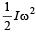and for ring I = mr2

Hence,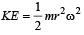QUESTION: 2

### A solid homogeneous sphere of mass M and radius R is moving on a rough horizontal surface, partly rolling and partly sliding. During this kind of motion of the sphere 

Solution:

Angular momentum a bout the point of contact with the surface includes the angular momentum about the centre. Due to friction linear momentum is conserved.

QUESTION: 3

### A solid cylinder of mass m and radius R rolls down an inclined plane of height h without slipping. The speed of its centre of mass when it reaches the bottom is 

Solution: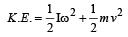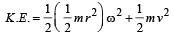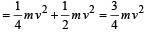Now, gain in K.E. = Loss in P.E.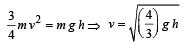QUESTION: 4

A particle of mass  m  is moving in a horizontal circle of radius  R  with uniform speed v. When it moves from one point to a diametrically opposite point, its 

Solution: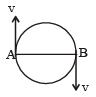At A, momentum = mv

Energy =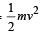At B, momentum = –mv

Energy =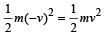Change in momentum = mv - (-mv) = 2mv

Change in energy =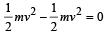QUESTION: 5

Moment of inertia of a uniform circular disc about a diameter is I. Its moment of inertia about an axis ⊥ to its plane and passing through a point on its rim will be 

Solution:

M.I of uniform circular disc about its diameter = I

According to theorem of perpendiclar axes, M.I. of disc about its axis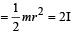Applying theorem of  | | axes,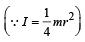M.I of disc about the given axis = 2 I + mr2 = 2 I + 4 I = 6 I

QUESTION: 6

The moment of inertia of a body about a given axis is 1.2 kg m2. Initially, the body is at rest. In order to produce a rotational kinetic energy of 1500 joule, an angular acceleration of 25  radian/sec2 must be applied about that axis for a duration of 

Solution: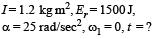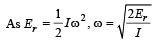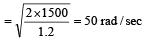From ω2 = ω1 + αt

50 = 0 + 25 t, t = 2 seconds

QUESTION: 7

A fly wheel rotating about a fixed axis has a kinetic energy of 360 joule when its angular speed is 30 radian/sec. The moment of inertia of the wheel about the axis of rotation is 

Solution: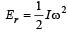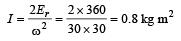QUESTION: 8

A particle of mass m = 5 is moving with a uniform speed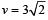in t h e XOY pl ane al o ng the line y = x + 4. The magnitude of the angular momentum of the particle about the origin is 

Solution: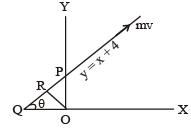y = x + 4 line has been shown in the figure when x = 0, y = 4. So, OP = 4.
The slope of  the line can be obtained by comparing with the equation of the straight line y = mx + c
m = tanθ = 1
⇒ θ = 45°

∠OQP = ∠OPQ = 45°
If we draw a line perpendicular to this line, length of the perpendicular = OR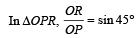⇒ OR = OP sin45°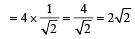Angular momentum of particle going along this line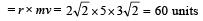QUESTION: 9

If a sphere is rolling , the ratio of the translational energy to total kinetic energy is given by 

Solution: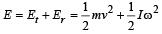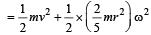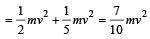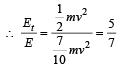QUESTION: 10

The speed of a homogenous solid sphere after rolling down an inclined plane of vertical height h from rest without sliding is 

Solution:

P.E. = total K.E.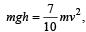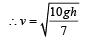QUESTION: 11

A solid  sphere, disc and solid cylinder all of the same mass and made of the same material are allowed to roll down (from rest ) on the inclined plane, then 

Solution:

For solid  sphere,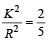For disc and solid cylinder,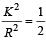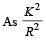for solid sphere is smallest, it takesminimum time to reach the bottom of the incline

QUESTION: 12

In a rectangle ABCD (BC = 2 AB). The moment of inertia is minimum along axis through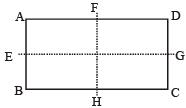Solution:

The M.I. is minimum about EG because mass distribution is at minimum distance from EG.

QUESTION: 13

Angular momentum is 

Solution:

Angular momentum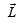is defined as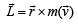So,is  an axial vector..

QUESTION: 14

A spherical ball rolls on a table without slipping.Then the fraction of its total energy associated with rotation is 

Solution: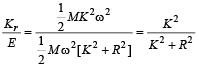Here,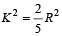QUESTION: 15

A thin uniform circular ring is rolling down an inclined plane of inclination  30° without slipping.
Its linear acceleration along the inclination plane will be 

Solution: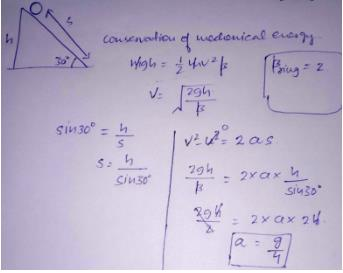QUESTION: 16

The angular speed of an engine wheel making 90 revolutions per minute is 

Solution:

Number of revolutions made by the engine wheel (n) = 90 per minute.
Angular speed of the engine wheel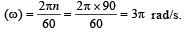QUESTION: 17

Two racing cars of masses m and 4m are moving in circles of radii r and 2r respectively. If their speeds are such that each makes a complete circle in the same time, then the ratio of the angular speeds of the first to the second car is 

Solution:

We know that both the cars take the same time to complete the circle, therefore ratio of angular speeds of the cars will be 1 : 1.

QUESTION: 18

The ABC is a triangular plate of uniform thickness. The sides are in the ratio shown in the figure. IAB, IBC and ICA are the moments of inertia of the piate about AB, BC and CA respectively. Which one of the following relations is correct ?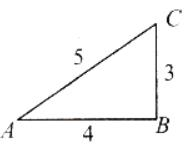Solution:

The intersection of medians is the centre of mass of the triangle. Since the distances of centre of mass from the sides is related as xBC < xAB < xAC Therefore  IBC  > IAB > IAC or   IBC  > IAB .

QUESTION: 19

If a flywheel makes 120 revolutions/minute, then its angular speed will be 

Solution:

Angular speed,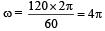rad/sec

QUESTION: 20

The angular momentum of a body with mass (m), moment of inertia (I) and angular velocity (ω) rad/sec is equal to 

Solution:

Let body contain m1, m2, m3 ........mn masses at distance r1, r2, r3 ........ rn from axis OA.
Angular momentum of body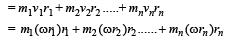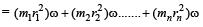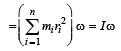QUESTION: 21

A couple produces 

Solution:

A couple is formed of two equal and opposite forces at some separation; so net force is zero. Hence ,a couple does not produce translatory motion; but it causes change in rotational motion.

QUESTION: 22

The centre of mass of a system of particles does not depend upon 

Solution:

Centre of mass of system depends upon position and masses of particle. Also, it depends upon relative distance between particles.

QUESTION: 23

In carbon monoxide molecule, the carbon and the oxygen atoms are separated by a distance 1.12 × 10–10 m. The distance of the centre of mass, from the carbon atom is 

Solution: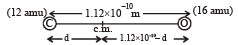From definition of centre of mass.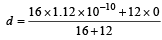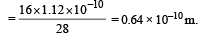QUESTION: 24

A thin circular ring of mass M and radius r is rotating about its axis with a constant angular velocity ω. Two objects each of mass m are attached gently to the opposite ends of a diameter of the ring.The ring will now rotate with an angular velocity 

Solution:

By the principle of conservation of angular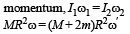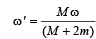QUESTION: 25

O is the centre of an equilateral triangle ABC. F1, F2 and F3 are three forces acting along the sides AB, BC and AC as shown here. What should be the magnitude of F3, so that the total torque about O is zero?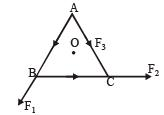Solution:

Perpendicular distance of each line of action of force from the centre is same, say x. Now taking moments about O,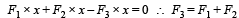QUESTION: 26

A weightless ladder 20 ft long rests against a frictionless wall at an angle of 60º from the horizontal. A 150 pound man is 4 ft from the top of the ladder. A horizontal force is needed to keep it from slipping. Choose the correct magnitude of the force from the following

Solution:

AB is the ladder, let F be the horizontal force and W is the weigth of man. Let N1 and N2 be normal reactions of ground and wall, respectively. Then for vertical equilibrium W = N1 .....(1)
For horizontal equilibrium, N2 = F .....(2)
Taking moments about A, N2(AB sin60°) – W(AC cos 60°) = 0 ......(3)
Using (2) and AB = 20ft, BC = 4 ft, we get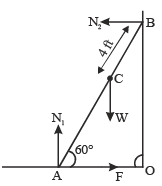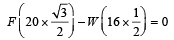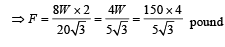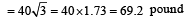QUESTION: 27

Two racing cars of masses m1 and m2 are moving in circles of radii r1 and r2 respectively. Their speeds are such that each makes a complete circle in the same time t. The ratio of the angular speeds of the first to the second car is 

Solution:

As time taken by both car to complete one revolution is same.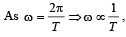as T is same in both

cases. Hence 'ω' will also be same.

QUESTION: 28

The moment of inertia of a disc of mass M and radius R about an axis, which is tangential to the circumference of the disc and parallel to its diameter, is 

Solution:

Moment of inertia of disc about its diameter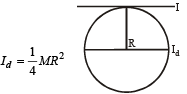MI of disc about a tangent passing through rim and in the plane of disc is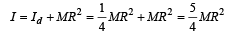QUESTION: 29

There is a flat uniform triangular plate ABC such that AB = 4 cm, BC = 3 cm and angle ABC = 90º.The moment of inertia of the plate about AB, BC and CA as axis is respectively I1, I2 and I3. Which one of the following is true?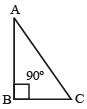Solution: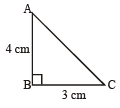Moment of Inertia depend upon mass and distribution of masses as  I = ∑mr2.
Further, as the distance of masses is more , more is the moment of Inertia.
If we choose BC as axis. Distance is maximum. Hence, Moment of Inertia is maximum.

∴ I2 > I1, I2 > I3

QUESTION: 30

A solid cylinder and a hollow cylinder both of the same mass and same external diameter are released from the same height at the same time on an inclined plane. Both roll down without slipping. Which one will reach the bottom first? 

Solution: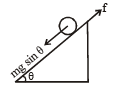Torque, Iα = f. R.
Using Newton's IInd law, mg sinα – f = ma

∵   pure rolling is there, a = Rα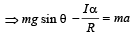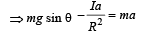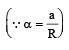or, acceleration,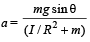Using,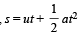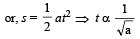t minimum means a should be more. This is possible when I is minimum which is the case for solid cylinder.
Therefore, solid cylinder will reach the bottom first.